# Vieta's formula and the roots of the polynomial

Definition: a Number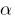is called a root of the polynomial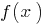, if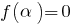(i.e.is a root of the equation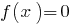). The number 3 is a root of the polynomial because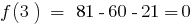## The elementary properties of roots

1. If the numberis a root of a polynomial, then this polynomial is divided by Declan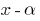without a trace — the consequence of Bézout's theorem;
2. Sinceis a root of the polynomial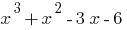then this polynomial is divided into;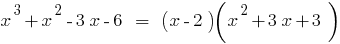3. A polynomial of degree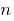has at mostroots;
4. If the polynomialit knowits roots: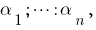then this polynomial can factorize: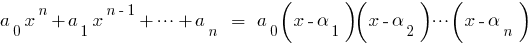.

## Formula Of Vieta

Ifthe roots of the polynomialthen comparing the coefficients of equal powerson the left and right, we obtain the relation between the roots of the polynomial and its coefficients, which are called formulas WTI.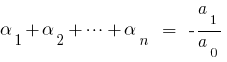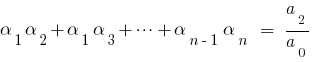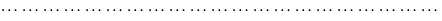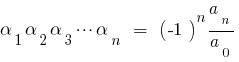When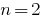for square trinomial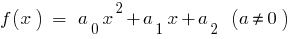are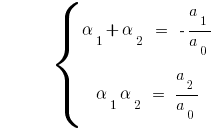If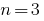for the cube of a trinomialareTags:
Chapter:
Versions in other languages:
Share with friends: